JEE  >  Test: Single Correct MCQs: Current Electricity | JEE Advanced

# Test: Single Correct MCQs: Current Electricity | JEE Advanced

Test Description

## 33 Questions MCQ Test Physics For JEE | Test: Single Correct MCQs: Current Electricity | JEE Advanced

Test: Single Correct MCQs: Current Electricity | JEE Advanced for JEE 2023 is part of Physics For JEE preparation. The Test: Single Correct MCQs: Current Electricity | JEE Advanced questions and answers have been prepared according to the JEE exam syllabus.The Test: Single Correct MCQs: Current Electricity | JEE Advanced MCQs are made for JEE 2023 Exam. Find important definitions, questions, notes, meanings, examples, exercises, MCQs and online tests for Test: Single Correct MCQs: Current Electricity | JEE Advanced below.
Solutions of Test: Single Correct MCQs: Current Electricity | JEE Advanced questions in English are available as part of our Physics For JEE for JEE & Test: Single Correct MCQs: Current Electricity | JEE Advanced solutions in Hindi for Physics For JEE course. Download more important topics, notes, lectures and mock test series for JEE Exam by signing up for free. Attempt Test: Single Correct MCQs: Current Electricity | JEE Advanced | 33 questions in 66 minutes | Mock test for JEE preparation | Free important questions MCQ to study Physics For JEE for JEE Exam | Download free PDF with solutions
 1 Crore+ students have signed up on EduRev. Have you?
Test: Single Correct MCQs: Current Electricity | JEE Advanced - Question 1

### The temperature coefficient of resistance of a wire is 0.00125 per °C. At 300 K, its resistance is 1 ohm. This resistance of the wire will be 2 ohm at.

Detailed Solution for Test: Single Correct MCQs: Current Electricity | JEE Advanced - Question 1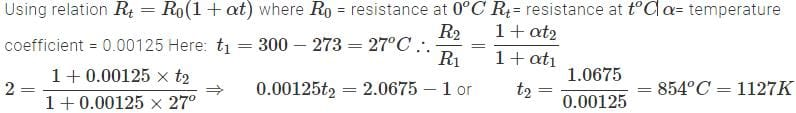Test: Single Correct MCQs: Current Electricity | JEE Advanced - Question 2

### A constant voltage is applied between the two ends of a uniform metallic wire. Some heat is developed in it. The heat developed is doubled if

Detailed Solution for Test: Single Correct MCQs: Current Electricity | JEE Advanced - Question 2

KEY CONCEPT : The heat produced is given by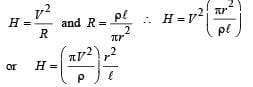Thus heat (H) is doubled if both length (l) and radius (r) are doubled.

Test: Single Correct MCQs: Current Electricity | JEE Advanced - Question 3

### The electrostatic field due to a point charge depends on the distance r as 1/r2 . Indicate which of the following quantities shows same dependence on r.

Detailed Solution for Test: Single Correct MCQs: Current Electricity | JEE Advanced - Question 3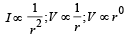Test: Single Correct MCQs: Current Electricity | JEE Advanced - Question 4

In the circuit shown in fig the heat produced in the 5 ohm resistor due to the current flowing through it is 10 calories per second.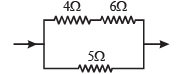The heat generated in the 4 ohms resistor is

Detailed Solution for Test: Single Correct MCQs: Current Electricity | JEE Advanced - Question 4

Since RAB = 2RCD therefore, current in AB will be half as compared to current in CD.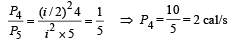Here P4 = Power dissipation in 4Ω

P5 = Power dissipation in 5Ω

Test: Single Correct MCQs: Current Electricity | JEE Advanced - Question 5

The current i in the circuit (see Fig) is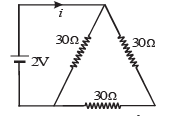Detailed Solution for Test: Single Correct MCQs: Current Electricity | JEE Advanced - Question 5

BC and AC are in series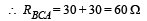Now BA and DC are in parallel.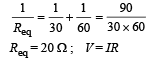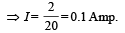Test: Single Correct MCQs: Current Electricity | JEE Advanced - Question 6

A piece of copper and another of germanium are cooled from room temperature to 80° K. The resistance of

Detailed Solution for Test: Single Correct MCQs: Current Electricity | JEE Advanced - Question 6

Copper is a metal whereas Germanium is Semi-conductor.
NOTE : Resistance of metal decreases and semiconductor increases with decrease in temperature.

Test: Single Correct MCQs: Current Electricity | JEE Advanced - Question 7

A battery of internal resistance 4Ω is connected to the network of resistances as shown. In order that the maximum power can be delivered to the network, the value of R in Ω should be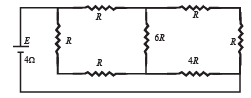Detailed Solution for Test: Single Correct MCQs: Current Electricity | JEE Advanced - Question 7

The equivalent circuits are shown in the figure.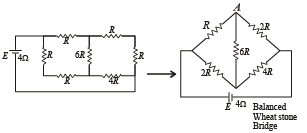The circuit represents balanced Wheatstone Bridge.
Hence 6R Ω resistance  is ineffective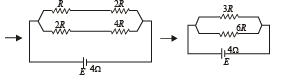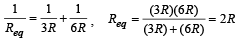For Max. Power External Resistance = Internal Resistance 2R = 4 Ω ∴ R = 2Ω

Test: Single Correct MCQs: Current Electricity | JEE Advanced - Question 8

In the  circuit P≠R , the reading of the galvanometer is same with switch S open or closed. Then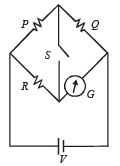Detailed Solution for Test: Single Correct MCQs: Current Electricity | JEE Advanced - Question 8

Since the opening or closing the switch does not affect the current through G, it means that in both the cases there is no current passing through S. Thus potential at A is equal to potential at B and it is the case of balanced wheatstone bridge..

IP = IQ and IR = IG

Test: Single Correct MCQs: Current Electricity | JEE Advanced - Question 9

In the given circuit, with steady current, the potential drop across the capacitor must be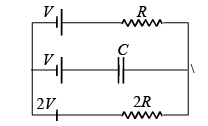Detailed Solution for Test: Single Correct MCQs: Current Electricity | JEE Advanced - Question 9

There will be no current flowing in branch BE in steady condition.
Let I be the current flowing in the loop ABCDEFA.
Applying Kirchoff's law in the loop moving in anticlockwise direction starting from C. + 2V – I (2R) – I (R) – V = 0
∴V = 3IR
⇒ I = V/3R ... (1)

Applying Kirchoff's law in the circuit ABEFA we get on moving  in anticlockwise direction starting from B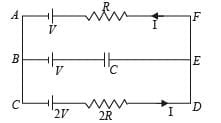+ V + Vcap – IR – V = 0 (where Vcap is the p.d. across capacitor).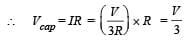Test: Single Correct MCQs: Current Electricity | JEE Advanced - Question 10

A wire of length L and 3 identical cells of negligible internal resistances are connected in series. Due to the current, the temperature of the wire is raised by ΔT in a time t. A number N of similar cells is now connected in series with a wire of the same material and cross-section but of length 2L. The temperature of the wire is raised by the same amount ΔT in the same time t. the value of N is

Detailed Solution for Test: Single Correct MCQs: Current Electricity | JEE Advanced - Question 10

Let R be the resistance of wire.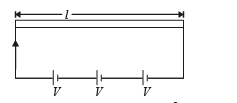Energy released in t second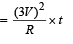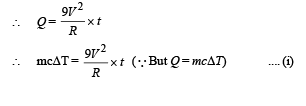Let R' be the resistance of the second wire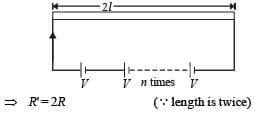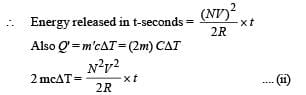Dividing (i) by (ii)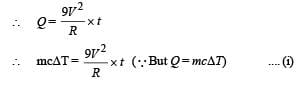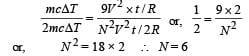or,        N 2 = 18 × 2    ∴ N = 6

Test: Single Correct MCQs: Current Electricity | JEE Advanced - Question 11

In the given circuit, it is observed that the current I is independent of the value of the resistance R6. Then the resistance values must satisfy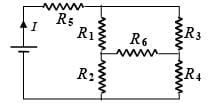Detailed Solution for Test: Single Correct MCQs: Current Electricity | JEE Advanced - Question 11

Since current I is independent of R6, it follows that the resistance R1, R2, R3 and R4 must form the balanced Wheatstone bridge.∴ R1 R4 = R2R3

Test: Single Correct MCQs: Current Electricity | JEE Advanced - Question 12

The effective resistance between points P and Q of the electrical circuit shown in the figure is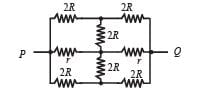Detailed Solution for Test: Single Correct MCQs: Current Electricity | JEE Advanced - Question 12

The circuit is symmetrical about the axis POQ.
The circuit above the axis POQ represents balanced wheatstone bride. Hence the central resistance 2R is ineffective. Similarly in the lower part (below the axis POQ) the central resistance 2R is ineffective.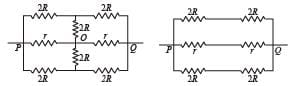Therefore the equivalent circuit is drawn.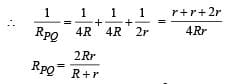Test: Single Correct MCQs: Current Electricity | JEE Advanced - Question 13

A 100 W bulb B1, and two 60 W bulb B2 an d B3, are connected to a 250 V source, as shown in figure. Now W1, W2 and W3 are the output powers of the bulbs B1, B2 and B3, respectively. Then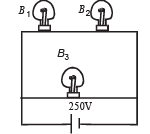Detailed Solution for Test: Single Correct MCQs: Current Electricity | JEE Advanced - Question 13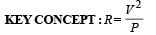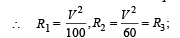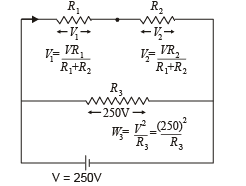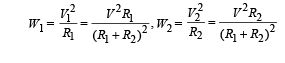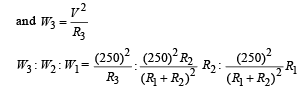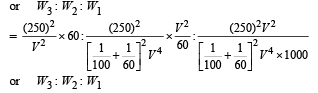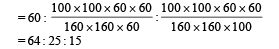Test: Single Correct MCQs: Current Electricity | JEE Advanced - Question 14

Express which of the following set ups can be used to verify Ohm’s law?

Detailed Solution for Test: Single Correct MCQs: Current Electricity | JEE Advanced - Question 14

In ohm's law, we check V = IR where I is the current flowing through a resistor and V is the potential difference across that resistor. Only option (a) fits the above criteria.
NOTE : Remember that ammeter is connected in series with resistance and voltmeter parallel with the net resistance.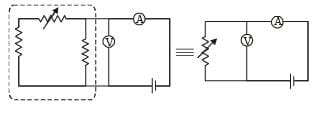Test: Single Correct MCQs: Current Electricity | JEE Advanced - Question 15

In the shown arrangement of the experiment of the meter bridge if AC corresponding to null deflection of galvanometer is x, what would be its value if the radius of the wire AB is doubled?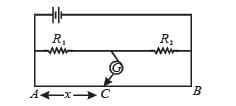Detailed Solution for Test: Single Correct MCQs: Current Electricity | JEE Advanced - Question 15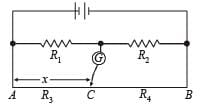At null point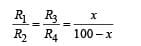If radius of the wire is doubled, then the resistance of AC will change and also the resistance of CB will change. But since R1/Rdoes not change so, R3/R4
should also not change at null point. Therefore the point C does not change.

Test: Single Correct MCQs: Current Electricity | JEE Advanced - Question 16

The three resistance of equal value are arranged in the different combinations shown below. Arrange them in increasing order of power dissipation.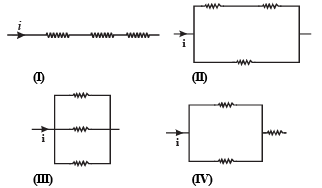Detailed Solution for Test: Single Correct MCQs: Current Electricity | JEE Advanced - Question 16

III < II < IV < I.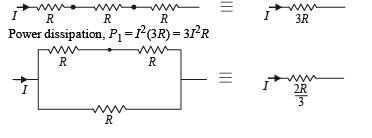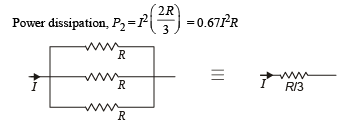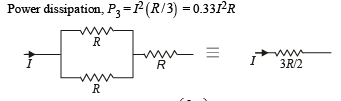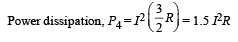Test: Single Correct MCQs: Current Electricity | JEE Advanced - Question 17

Shown in figure is a Post Office box. In order to calculate the value of external resistance, it should be connected between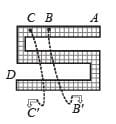Detailed Solution for Test: Single Correct MCQs: Current Electricity | JEE Advanced - Question 17

Total external resistance will be the total resistance of whole length of box. It should be connected between A and D.

Test: Single Correct MCQs: Current Electricity | JEE Advanced - Question 18

Six identical resistors are connected as shown in the figure.The equivalent resistance will be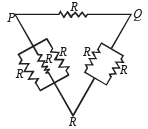Detailed Solution for Test: Single Correct MCQs: Current Electricity | JEE Advanced - Question 18

For var ious combination s equivalent r esistan ce is maximum between P and Q.

Test: Single Correct MCQs: Current Electricity | JEE Advanced - Question 19

A capacitor is charged using an exter nal batter y with a resistance x in series. The dashed line shows the variation of ln I with respect to time. If the resistance is changed to 2x, the new graph will be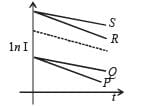Detailed Solution for Test: Single Correct MCQs: Current Electricity | JEE Advanced - Question 19

KEY CONCEPT : The current in RC circuit is given by I = I0e–t/RC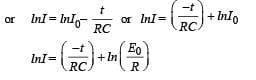On comparing with y = mx + C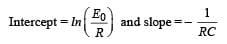When R is changed to 2R then slope increases and current becomes less. New graph is Q.

Test: Single Correct MCQs: Current Electricity | JEE Advanced - Question 20

Find out the value of current through 2Ω resistance for the given circuit.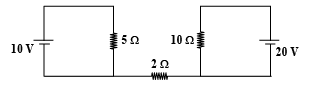Detailed Solution for Test: Single Correct MCQs: Current Electricity | JEE Advanced - Question 20

The current in 2W resistor will be zero because it is not a part of any closed loop.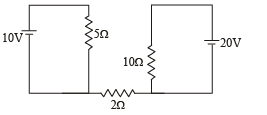Test: Single Correct MCQs: Current Electricity | JEE Advanced - Question 21

A 4 μF capacitor, a resistance of 2.5 MΩ is in series with 12 V battery. Find the time after  which the potential difference across the capacitor is 3 times the potential difference across the resistor. [Given ln(2) = 0.693]

Detailed Solution for Test: Single Correct MCQs: Current Electricity | JEE Advanced - Question 21

KEY CONCEPT : At any instant of time t during charging process, the transient current in the circuit is given by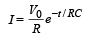∴ Potential difference across resistor R is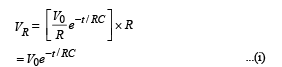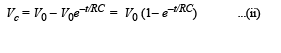∴ Potential diff. across C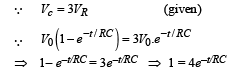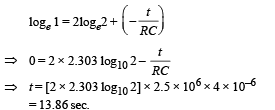Test: Single Correct MCQs: Current Electricity | JEE Advanced - Question 22

A moving coil galvanometer of resistance 100 Ω is used as an ammeter using a resistance 0.1 Ω.  The maximum deflection current in the galvanometer is 100 μA. Find the minimum current in the circuit so that the ammeter shows maximum deflection

Detailed Solution for Test: Single Correct MCQs: Current Electricity | JEE Advanced - Question 22

KEY CONCEPT :

IgG = (I – Ig) S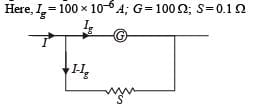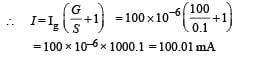Test: Single Correct MCQs: Current Electricity | JEE Advanced - Question 23

An ideal gas is filled in a closed rigid and thermally insulated container. A coil of 100 Ω resistor carrying current 1 A for 5 minutes supplies heat to the gas. The change in internal energy of the gas is

Detailed Solution for Test: Single Correct MCQs: Current Electricity | JEE Advanced - Question 23

The heat supplied under these conditions is the change in internal energy Q = ΔU
The heat supplied Q = i2RT = 1 × 1 × 100 × 5 × 60 = 30,000 J = 30 kJ

Test: Single Correct MCQs: Current Electricity | JEE Advanced - Question 24

If a steady current I is flowing through a cylindrical element ABC. Choose the correct relationship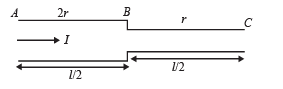Detailed Solution for Test: Single Correct MCQs: Current Electricity | JEE Advanced - Question 24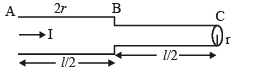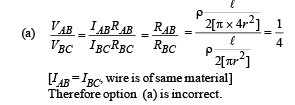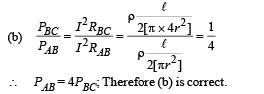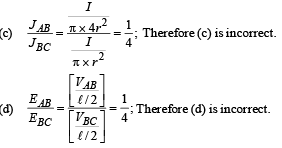Test: Single Correct MCQs: Current Electricity | JEE Advanced - Question 25

A resistance of 2Ω is connected across one gap of a metrebridge (the length of the wire is 100 cm) and an unknown resistance, greater than 2Ω, is connected across the other gap. When these resistances are interchanged, the balance point shifts by 20 cm. Neglecting any corrections, the unknown resistance is

Detailed Solution for Test: Single Correct MCQs: Current Electricity | JEE Advanced - Question 25

Given X is greater than 2Ω when the bridge is balanced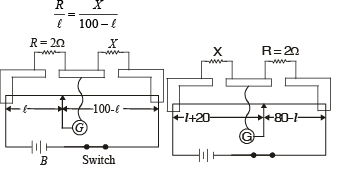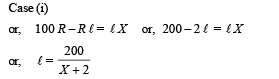When the resistances are interchanged the jockey shifts 20 cm. Therefore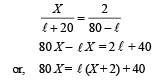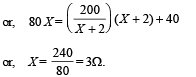Test: Single Correct MCQs: Current Electricity | JEE Advanced - Question 26

A circuit is connected as shown in the figure with the switch S open. When the switch is closed, the total amount of charge that flows from Y to X is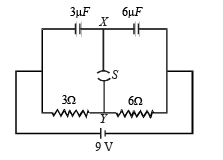Detailed Solution for Test: Single Correct MCQs: Current Electricity | JEE Advanced - Question 26

The total charge enclosed in the dotted portion when the switch S is open is zero. When the switch is closed and steady state is reached, the current I coming from the battery is 9 = I (3 + 6) ⇒ I = 1A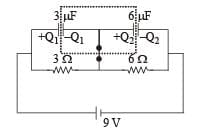∴ Potential difference across 3Ω resistance = 3V and potential difference across 6Ω resistance = 6V

∴ p.d. across 3 µF capacitor = 3V an d p.d. across 6 µF capacitor = 6V

∴ Charge on 3 µF capacitor Q1 = 3 × 3 = 9 µC Charge on 6 µF capacitor Q2 = 6 × 6 = 36 µC The total charge enclosed in the dotted portion =

∴ Charge passing the switch = 36 – 9 = 27 µC

Test: Single Correct MCQs: Current Electricity | JEE Advanced - Question 27

Figure shows three resistor configurations R1, R2 and R3 connected to 3V battery. If the power dissipated by the configuration R1, R2 and R3, is P1, P2 and P3, respectively, then –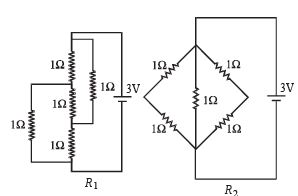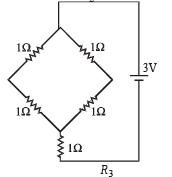Detailed Solution for Test: Single Correct MCQs: Current Electricity | JEE Advanced - Question 27

We know that
P=We know that
P=V2/R
For constant value of potential difference (V) we have
For constant value of potential difference (V) we have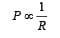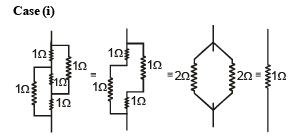This is a case of balanced Wheatstone bridge R1 = 1Ω Case (ii)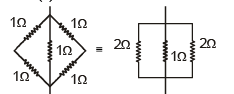Clearly the equivalent resistance (R2) will be less than 1Ω.
Case (iii)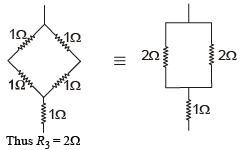Since, R2 < R1 < R3

∴ P2 > P1 > P3

Test: Single Correct MCQs: Current Electricity | JEE Advanced - Question 28

Incandescent bulbs are designed by keeping in mind that the resistance of their filament increases with the increase in temperature. If at room temperature, 100 W, 60 W and 40 W bulbs have filament resistances R100, R60 and R40, respectively, the relation between these resistances is

Detailed Solution for Test: Single Correct MCQs: Current Electricity | JEE Advanced - Question 28

We know that P=V2/R

For a given potential difference at a particular temperature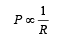It is given that the powers of the bulbs are in the order

100W > 60 W > 40W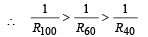Test: Single Correct MCQs: Current Electricity | JEE Advanced - Question 29

To verify Ohm’s law, a student is provided with a test resistor RT, a high resistance R1, a small resistance R2, two identical galvanometers G1 and G2, and a variable voltage source V. The correct circuit to carry out the experiment is

Detailed Solution for Test: Single Correct MCQs: Current Electricity | JEE Advanced - Question 29

c) The following points should be considered while making the circuit :

(i) An ammeter is made by connecting a low resistance R2 in parallel with the galvanometer G2.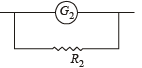(ii) A voltmeter is made by connecting a high resistance R1 in series with the galvanometer G1.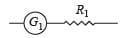(iii) Voltmeter is connected in parallel with the test resistor RT.

iv) Ammeter is connected in series with the test resistor RT.
(v) A variable voltage source V is connected in series with the test resistor RT.

Test: Single Correct MCQs: Current Electricity | JEE Advanced - Question 30

Consider a thin square sheet of side L and thickness t, made of a material of resistivity ρ. The resistance between two opposite faces, shown by the shaded areas in the figure is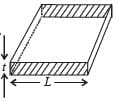Detailed Solution for Test: Single Correct MCQs: Current Electricity | JEE Advanced - Question 30

We know that R=ρ l/a

Where l is the length of the conductor through which the current flows and a is the area of cross section.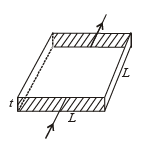Here l = L and a = L × t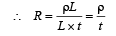∴ R is independent of L

Test: Single Correct MCQs: Current Electricity | JEE Advanced - Question 31

A meter bridge is set up as shown, to determine an unknown resistance ‘X’ using a standard 10 ohm resistor. The galvanometer shows null point when tapping-key is at 52 cm mark. The end-corrections are 1 cm and 2 cm respectively for the ends A and B. The determined value of ‘X’ is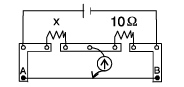Detailed Solution for Test: Single Correct MCQs: Current Electricity | JEE Advanced - Question 31

At Null point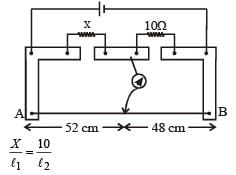Here l1 = 52 + End correction = 52 + 1 = 53 cm

l2 = 48 + End correction = 48 + 2 = 50 cm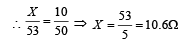Test: Single Correct MCQs: Current Electricity | JEE Advanced - Question 32

During an experiment with a metre bridge, the galvanometer shows a null point when the jockey is pressed at 40.0 cm using a standard resistance of 90 Ω, as shown in the figure.  The least count of the scale used in the metre bridge is 1mm. The unknown resistance is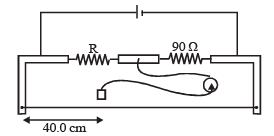Detailed Solution for Test: Single Correct MCQs: Current Electricity | JEE Advanced - Question 32

In case of a meter bridge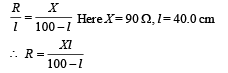For finding the value of R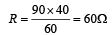For finding the value of ΔR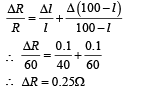Therefore, R = (60 ± 0.25)Ω

Test: Single Correct MCQs: Current Electricity | JEE Advanced - Question 33

An infinite line charge of uniform electric charge density λ lies along the axis of an electrically conducting infinite cylindrical shell of radius R. At time t = 0, the space inside the cylinder is filled with a material of permittivity ε and electrical conductivity σ. The electrical conduction in the material follows Ohm's law. Which one of the following graphs best describes the subsequent variation of the magnitude of current density j(t) at any point in the material?

Detailed Solution for Test: Single Correct MCQs: Current Electricity | JEE Advanced - Question 33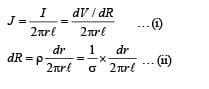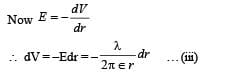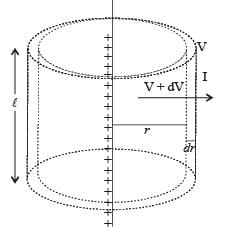From (i), (ii), and (iii)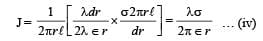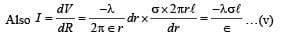Here negative sign signifies that the current is decreasing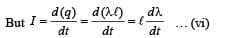From (v) and (vi)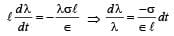On integrating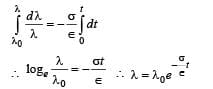Substituting this value in (iv) we get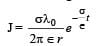## Physics For JEE

257 videos|633 docs|256 tests
 Use Code STAYHOME200 and get INR 200 additional OFF Use Coupon Code
Information about Test: Single Correct MCQs: Current Electricity | JEE Advanced Page
In this test you can find the Exam questions for Test: Single Correct MCQs: Current Electricity | JEE Advanced solved & explained in the simplest way possible. Besides giving Questions and answers for Test: Single Correct MCQs: Current Electricity | JEE Advanced, EduRev gives you an ample number of Online tests for practice

## Physics For JEE

257 videos|633 docs|256 tests